# PCA revisited

August 12, 2018
By

Want to share your content on R-bloggers? click here if you have a blog, or here if you don't.

Principal component analysis (PCA) is a dimensionality reduction technique which might come handy when building a predictive model or in the exploratory phase of your data analysis. It is often the case that when it is most handy you might have forgot it exists but let’s neglect this aspect for now 😉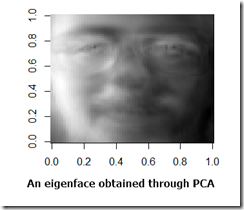I decided to write this post mainly for two reasons:

1. I had to make order in my mind about the terminology used and complain about a few things.
2. I wanted to try to use PCA in a meaningful example.

## What does it do?

There are a lot of ways to try to explain what PCA does and there are a lot of good explanations online. I highly suggest you to google around a bit on the topic.

PCA looks for a new the reference system to describe your data. This new reference system is designed in such a way to maximize the variance of the data across the new axis. The first principal component accounts for as much variance as possible, as does the second and so on. PCA transforms a set of (tipically) correlated variables into a set of uncorrelated variables called principal components. By design, each principal component will account for as much variance as possible. The hope is that a fewer number of PCs can be used to summarise the whole dataset. Note that PCs are a linear combination of the original data.

The procedure simply boils down to the following steps

1. Scale (normalize) the data (not necessary but suggested especially when variables are not homogeneous).
2. Calculate the covariance matrix of the data.
3. Calculate eigenvectors (also, perhaps confusingly, called “loadings”) and eigenvalues of the covariance matrix.
4. Choose only the first N biggest eigenvalues according to one of the many criteria available in the literature.
5. Project your data in the new frame of reference by multipliying your data matrix by a matrix whose columns are the N eigenvectors associated with the N biggest eigenvalues.
6. Use the projected data (very confusingly called “scores”) as your new variables for further analysis.

Everything clear? No? Ok don’t worry, it takes a bit of time to sink in. Check the practical example below.

## Use case: why should you use it?

The best use cases I can find at the top of my mind are the following:

1. Redundant data: you have gathered a lot of data on a system and you suspect that most of that data is not necessary to describe it. Example: a point moving on a straight line with some noise, say X axis, but you somehow have collected data also on Y and Z axis. Note that PCA doesn’t get rid of the redundant data, it simply costructs new variables that summarise the original data.
2. Noise in your data: the data you have collected is noisy and there are some correlations that might annoy you later
3. The data is heavily redundant and correlated. You think out of the 300 variables you have, 3 might be enough to describe your system.

## Use case: when should you use it?

PCA is a technique used in the exploratory phase of data analysis. Personally, I would use it only in one of the 3 circumstances above, at the beginning of my analisis, after having confirmed through the basic descriptive statistics tools and some field knowledge, that I am indeed in one of the use cases mentioned. I would also probably use it when trying to improve a model that relies on independence of the variables.

## Not use case: when should you not use it?

PCA is useless when your data is mostly indipendent or even when you have very few variables I would argue. Personally, I would not use it when a clean and direct interpretation of the model is required either. PCA is not a black box but its output is difficult to a) explain to non technical people and, in general, b) not easy to interpret.

## Example of application: the Olivetti faces dataset

The Olivetti faces dataset is a collection of 64×64 greyscale pixel images of 40 faces, there are 10 images for each face. It is available in Scikit-learn and it is a good example dataset for showing what PCA can do. I think it is a better cheap example than the iris dataset since it can show you why you would use PCA.

Then, we need to shape the data in a way that is acceptable for our analysis, that is, features (also known as variables) should be columns, and samples (also known as observations) should be the rows. In our case the data matrix should have size 399×4096. Row 1 would represent image 1, row 2 image 2, and so on… To achieve this, I used this simple R script with a for loop

The images in the dataset are all head shots with mostly no angle. This is an example of the first face (label 0)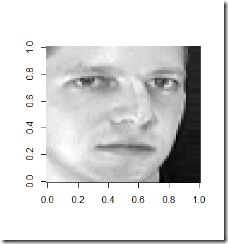A very simple thing to do would be to look what the average face looks like. We can do this by using dplyr, grouping by label and then taking the average of each pixel. Below you can see the average face for labels 0, 1 and 2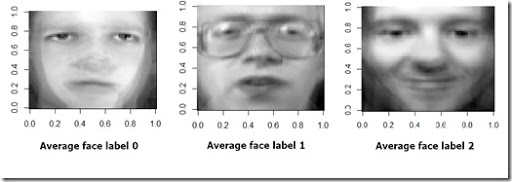Then it is time to actually applying PCA on the dataset

Since the covariance matrix has dimensions 4096×4096 and it is real and symmetric, we get 4096 eigenvalues and 4096 corresponding eigenvectors. I decided (after a few trials) to keep only the first 20 which account roughly for 76.5 % of the total variance in the dataset. As you can see from the plot below, the magnitude of the eigenvalues goes down pretty quickly.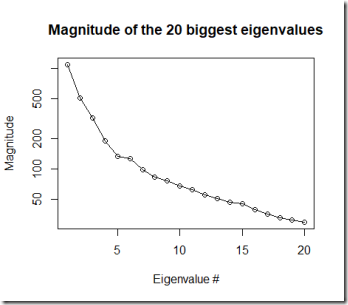A few notes:

1. The eigenvalues of the covariance matrix represent the variance of the principal components.
2. Total variance is preserved after a rotation (i.e. what PCA is at its core).
3. The variable D_new contains the new variables in the reduced feature space. Note that by using only 20 variables (the 20 principal components) we can capture 76.5 % of the total variance in the dataset. This is quite remarkable since we can probably avoid using all the 4096 pixels for every image. This is good news and it means that PCA application in this case might be useful! If I had to use 4000 principal components to capture a decent amount of variance, that would have been a hint that PCA wasn’t probably the right tool for the job. This point is perhaps trivial, but particularly important. One must not forget what each tool is designed to accomplish.
4. There are several methods for choosing how many principal components should be kept.  I simply chose 20 since it seems to work out fine. In a real application you might want to research a more scientific criterion or make a few trials.

## The eigenfaces

What is an eigenface? The eigenvectors of the covariance matrix are the eigenfaces of our dataset. These face images form a set of basis features that can be linearly combined to reconstruct images in the dataset. They are the basic characteristic of a face (a face contained in this dataset of course) and every face in this dataset can be considered to be a combination of these standard faces. Of course this concept can be applied also to images other than faces. Have a look at the first 4 (and the 20th) eigenfaces. Note that the 20th eigenface is a bit less detailed than the first 4. If you were to look at the 4000 eigenface, you would find that it is almost white noise.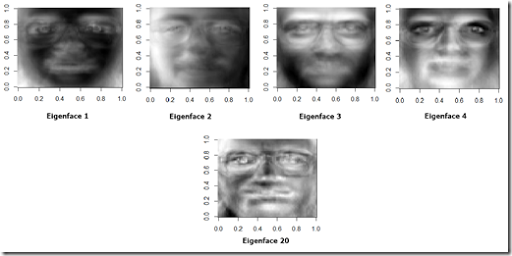Then we can project the average face onto the eigenvector space. We can do this also for a new image and use this new data, for instance, to do a classification task.

This is the plot we obtain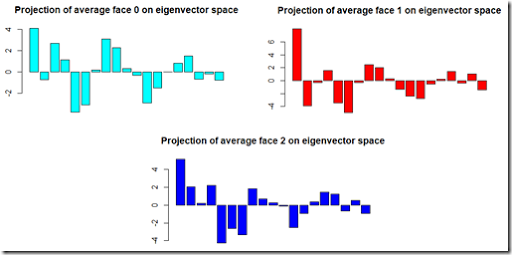As you can see, the 3 average faces rank quite differently in the eigenvectors’ space. We could use the projections of a new image to perform a classification task on an algorithm trained on a database of images transformed according to PCA. By using only a few principal components we could save computational time and speed up our face recognition (or other) algorithm.

## Using R built-in prcomp function

For the first few times, I would highly suggest you to do PCA manually, as I did above, in order to get a firm grasp at what you are actually doing with your data. Then, once you are familiar with the procedure, you can simply use R built-in prcomp function (or any other that you like) which, however,  has the downside of calculating all the eigenvalues and eigenvectors and therefore can be a bit slow on a large dataset. Below you can find how it can be done.

I hope this post was useful.

R-bloggers.com offers daily e-mail updates about R news and tutorials about learning R and many other topics. Click here if you're looking to post or find an R/data-science job.
Want to share your content on R-bloggers? click here if you have a blog, or here if you don't.